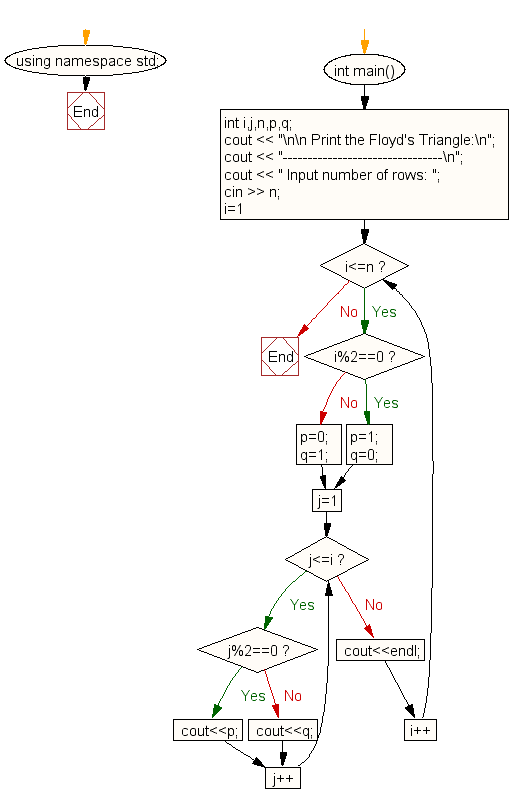﻿ C++ Exercises: Print the Floyd's Triangle - w3resource# C++ Exercises: Print the Floyd's Triangle

## C++ For Loop: Exercise-43 with Solution

Write a program in C++ to print the Floyd's Triangle.

Sample Solution:-

C++ Code :

``````#include <iostream>
using namespace std;

int main()
{
int i,j,n,p,q;
cout << "\n\n Print the Floyd's Triangle:\n";
cout << "--------------------------------\n";
cout << " Input number of rows: ";
cin >> n;
for(i=1;i<=n;i++)
{
if(i%2==0)
{
p=1;q=0;
}
else
{
p=0;q=1;
}
for(j=1;j<=i;j++)
if(j%2==0)
cout<<p;
else
cout<<q;
cout<<endl;
}
}
``````

Sample Output:

``` Print the Floyd's Triangle:
--------------------------------
Input number of rows: 5
1
01
101
0101
10101
```

Flowchart:C++ Code Editor:

Contribute your code and comments through Disqus.

What is the difficulty level of this exercise?

﻿

## C++ Programming: Tips of the Day

What is a smart pointer and when should I use one?

This answer is rather old, and so describes what was 'good' at the time, which was smart pointers provided by the Boost library. Since C++11, the standard library has provided sufficient smart pointers types, and so you should favour the use of std::unique_ptr, std::shared_ptr and std::weak_ptr.

There was also std::auto_ptr. It was very much like a scoped pointer, except that it also had the "special" dangerous ability to be copied - which also unexpectedly transfers ownership.

It was deprecated in C++11 and removed in C++17, so you shouldn't use it.

```std::auto_ptr<MyObject> p1 (new MyObject());
std::auto_ptr<MyObject> p2 = p1; // Copy and transfer ownership.
// p1 gets set to empty!
p2->DoSomething(); // Works.
p1->DoSomething(); // Oh oh. Hopefully raises some NULL pointer exception.
```

Ref : https://bit.ly/3mc9GHE